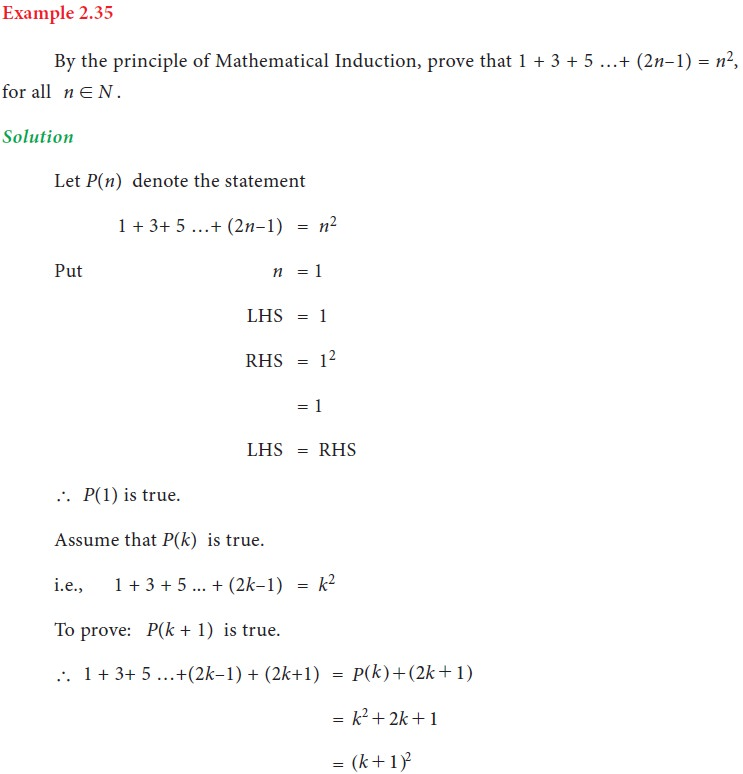Home | | Business Maths 11th std | Mathematical induction

# Mathematical induction

Mathematical induction is one of the techniques which can be used to prove variety of mathematical statements which are formulated in terms of n, where n is a positive integer.

Mathematical induction
Mathematical induction is one of the techniques which can be used to prove variety of mathematical statements which are formulated in terms of n, where n is a positive integer.
Mathematical Induction is used in the branches of Algebra, Geometry and Analysis where it turns out to be necessary to prove some truths of propositions.Tags : Definition, Formula, Solved Example Problems, Exercise | Mathematics , 11th Business Mathematics and Statistics(EMS) : Chapter 2 : Algebra
Study Material, Lecturing Notes, Assignment, Reference, Wiki description explanation, brief detail
11th Business Mathematics and Statistics(EMS) : Chapter 2 : Algebra : Mathematical induction | Definition, Formula, Solved Example Problems, Exercise | Mathematics